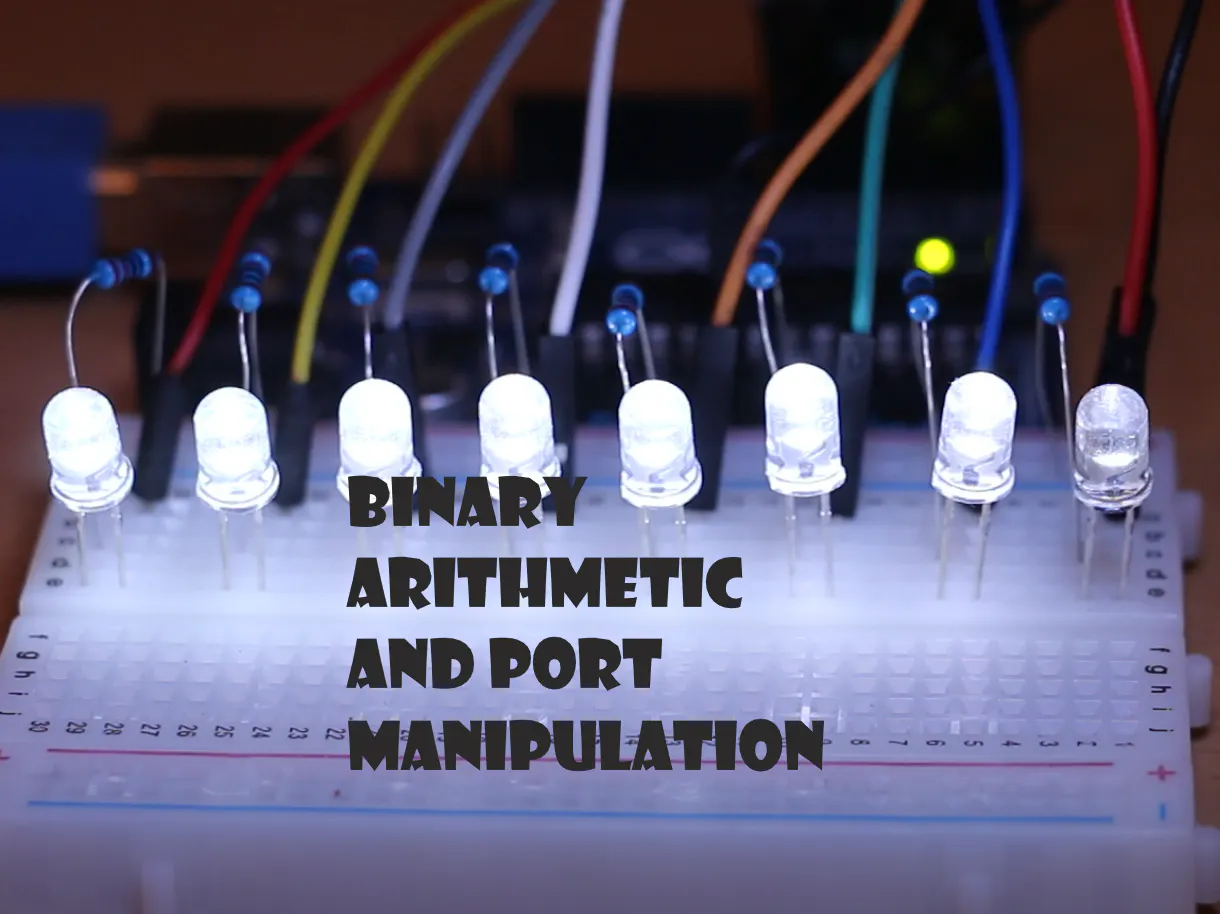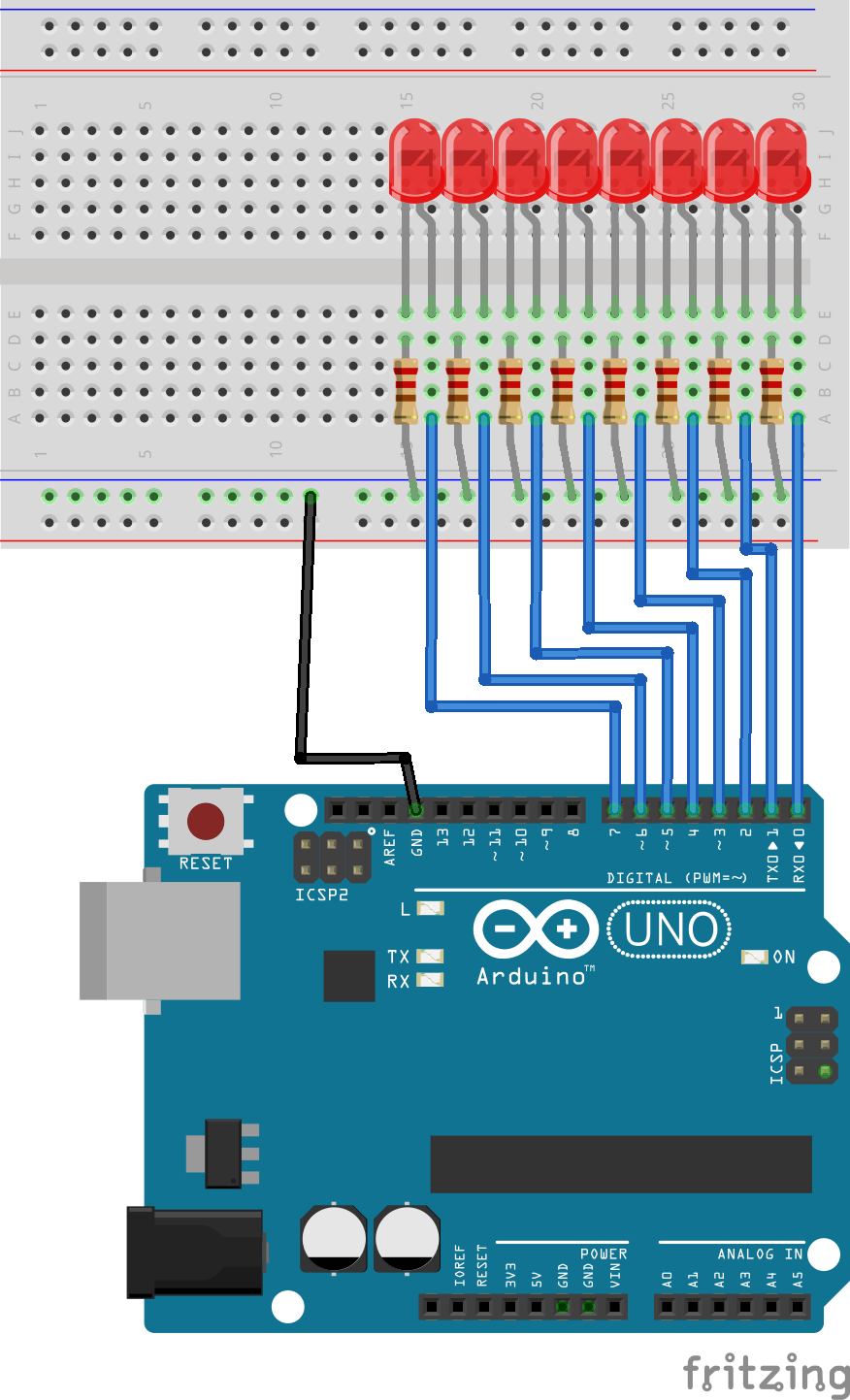# Binary Arithmetic and Port Manipulation © GPL3+

One port to role them all.

• 7,270 views
• 37 respects

## Apps and online services

In this tutorial I will cover the basics of binary arithmetic and its practical uses in arduino.

Setting and storing the state of 8 leds, with only one byte of data.

Also covering some of the less known and used bit functions of Arduino, like bitSet, bitClear and some other bit functions.

For more read about binary arithmetic and its practical uses in arduino

https://playground.arduino.cc/Code/BitMath

https://www.arduino.cc/en/Reference/PortManipulation

## Code

##### The finished example codeArduino
Show the steps (commented out) before using the port
```//boolean ledState = {0, 0, 0, 0, 0, 0, 0, 0};
byte ledState = 0;

void setup() {
//  for (int i = 0; i < 8; i++) {
//    pinMode(i, OUTPUT);
//  }
DDRD = B11111111;
}

void loop() {
for (int i = 0; i < 8; i++) {
//digitalWrite(i,HIGH);
//ledState[i] = HIGH;
bitSet(ledState, i);
delay(100);

}
for (int i = 0; i < 8; i++) {
//digitalWrite(i,LOW);
//ledState[i] = LOW;
bitClear(ledState, i);
delay(100);
}
}

//  for (int i = 0; i < 8; i++) {
//    //digitalWrite(i,ledState );
//  }
PORTD  = ledState;
}
```

## Schematics#### Binary Arithmetic and Port Manipulation – Part II

Project tutorial by Tal O

• 1,328 views
• 3 respects

• 55,147 views
• 48 respects

#### 3-Bit Binary Calculator Using Arduino Uno

Project showcase by 22warehamD

• 11,356 views
• 17 respects

#### Binary Wristwatch

Project showcase by thallia

• 3,152 views
• 12 respects

#### Digital And Binary Clock With Two LED Matrix And RTC

Project showcase by LAGSILVA

• 21,526 views